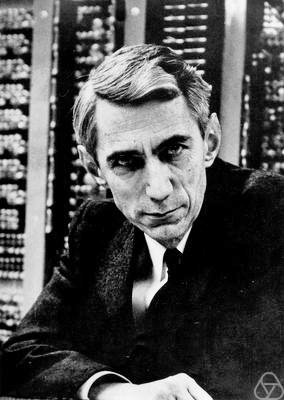# Happy Birthday, Claude Shannon

Claude Shannon is famous among mathematicians and computer scientists for his remarkable work, particularly in the realm of  information theory.  Of particular importance is Shannon’s notion of information entropy, often referred to now as “Shannon entropy”.  He launched the theory in 1948 in a remarkable paper titled, “A Mathematical Theory of Communication“.   Below, I will reproduce Joseph Doob‘s review of Shannon’s famous paper.Photo credit: Oberwolfach Photo Collection (CC BY-SA 2.0 DE)

During World War II, he worked briefly with Alan Turing, when Turing came to the United States to advise the American crypto efforts.  Shannon had a playful streak, too.  He was an avid juggler, and enjoyed unicycles.  Shannon was fond of toys.  He constructed a version of Marvin Minsky‘s useless machine, a device whose only function is to turn itself off.  There is a great video of one such machine available.   Siobhan Roberts has an excellent profile of Shannon in the New Yorker.  Other tributes abound, of course.

MR0026286
Shannon, C. E.
A mathematical theory of communication.
Bell System Tech. J. 27, (1948). 379–423, 623–656.
60.0X

The author considers an information source which can produce any one of a finite number of symbols. These are fed into a channel for transmission, each symbol having a positive time duration in the channel. The problem becomes statistical with the assumption that if $x_n$ is the $n$th symbol produced by the source the $x_n$ process is a stationary stochastic process. (Further hypotheses of Markov type are also made in the applications.) The concept of the entropy $H(U)$ of a random variable $U$ which can assume only a finite number of values, with probabilities $p_1,p_2,cdots$, is fundamental: $H(U)=-sum_ip_ilog p_i$. The entropy is a measure of the uncertainty in the value of $U$, and has various suggestive properties indicating this; for example $H(U)$ is greater than or equal to the entropy of $U$ conditioned by the knowledge of a second random variable. If the $x_n$‘s above are mutually independent the entropy of the information source is defined as $H(x_n)$, and is a measure of the amount of information available in the source for transmission. A slightly modified definition of source entropy is made if the $x_n$‘s are not mutually independent. The capacity of a channel is defined as $C=lim_{Trightarrowinfty}log N(T)/T$, where $N(T)$ is the maximum number of sequences of symbols that can be transmitted in time $T$, and it is shown that for given $H$ and $C$ the symbols can be encoded in such a way that $C/H-epsilon$ symbols per second can be transmitted over the channel if $epsilon>0$ but not if $epsilon<0$. Many examples are given and the effect of noise on the transmission is treated in some detail. For example, if $Hleq C$ above, there exists a coding system such that the output of the source can be transmitted over the channel with an arbitrary small error frequency. [For the early beginnings of this theory cf. Nyquist, same J. 3, 324–346 (1924); Hartley, ibid. 7, 535–563 (1928).]

In the second part of the paper the author treats absolutely continuous distributions. The entropy of a distribution in one or more dimensions, with density $p(x)$, is defined as $-int p(x)log p(x),dx$ and various qualitative properties of entropy are discussed. For example, the entropy of a distribution in one dimension, with finite dispersion $sigma^2$, is a maximum (relative to a not very clearly specified set of unbounded distributions) for a normal distribution. The entropy per degree of freedom of a stationary and ergodic process with random variables $x_1,x_2,cdots$ is defined as $H’=lim_{nrightarrowinfty}H_n/n$, where $H_n$ is the entropy of the distribution of $x_1,cdots,x_n$, and it is stated that if $p(x_1,cdots,x_n)$ is the density of the latter distribution, then $n^{-1}log p$ converges in probability to $H’$. The entropy decrease caused by the action of a linear filter on a stationary ergodic process is evaluated. The rate $R$ of transmission of information over a channel is defined and the channel capacity is then defined as the maximum of $R$ for an arbitrarily varying input. For example the capacity of a channel of band $0$to $W$ cps perturbed by white noise of power $N$, for average transmission power $P$, is $Wlog(P+N)/P$ and this can be interpreted to mean that proper coding will allow the transmission of this many binary digits of information per second with arbitrarily small error frequency. Finally the rate of generating information relative to a certain degree of fidelity is defined and discussed. The discussion is suggestive throughout, rather than mathematical, and it is not always clear that the author’s mathematical intentions are honorable. The point of view is that stressed by Wiener in his NDRC report [soon to be published as a book] “The Interpolation, Extrapolation, and Smoothing of Stationary Time Series,” in which communication is considered as a statistical problem, specifically in its mathematical formulation as the study of stationary stochastic processes, and of the results of various operations performed on them.

Reviewed by J. L. Doob